### 3. LOCAL THEORY OF DENSITY WAVES

Since solutions of Poisson's equation can be obtained analytically only for the simplest mass distributions, theoretical treatments necessarily make quite drastic simplifying assumptions. Local theory, summarized in this section, is built around a WKB-type potential solution for density variations in the form of short wavelength plane waves in a locally uniform, razor thin disk. In this case, a plane-wave disturbance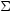1 eikx to the surface density in the (x, y)-plane at some instant gives rise to the potential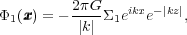(7)

(cf. eq. 6.31 of BT08). This relation strictly applies only to straight waves of infinite extent. However, the sinusoidal density variations cause the field of distant waves to cancel quickly, and the formula is reasonably accurate near the center of a short wave packet. Note that eq. (7) does not depend on the inclination of the wavefronts to the radial direction, but the curvature of the wavefronts must also be negligible, which requires |kR| >> 1, with R being the distance from the disk center.

A dispersion relation gives the relationship between the wavenumber k and frequencyof self-consistent waves. Assuming (a) the above relation between density and potential for a razor-thin disk and (b) the wave is of small amplitude, so that second order terms are negligible, the local dispersion relation for short axisymmetric density waves may be written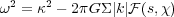(8)

(Kalnajs 1965), whereis the local undisturbed surface density and the complicated reduction factor, with two dimensionless arguments, is explained below. Lin & Shu (1966) independently derived a generalized relation for tightly-wrapped spiral waves, which required two additional assumptions: (c) that the wave vector k is approximately radial, and (d) that the disturbance is not close to any of the principal resonances. Their better-known relation simply replaces the frequency of the wave with the Doppler-shifted forcing frequency experienced by the orbit guiding center: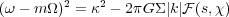(9)

(see also BT08 Section 6.2.2), where nowmp.

The reduction factor,, is always positive and is unity when the disk has no random motion. Since all the factors in the self-gravity term on the RHS are intrinsically positive, eqs. (8) & (9) say that self-gravity enables a supporting response from the stars at a frequency that is lower than their free epicycle frequency.

When the disk has random motion, the vigor of the stellar response depends on two factors: the ratio of the forcing frequency experienced by a star to its natural frequency, s|- m| /, and the ratio of the typical sizes of the stellar epicycles (< vR2 >1/2 /) to the wavelength of the wave (|k|-1). Thus for a Gaussian distribution of radial velocities,is a function of both s andR2 k2 /2. Clearly, whenis large, most stars will oscillate over many radial waves, and the weakened supporting response arises mainly from the small fraction of stars near the center of the velocity distribution.

The dispersion relation for barotropic fluid (gaseous) disks 2 is similar (BT08 eq. 6.55)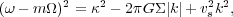(10)

where vs is the sound speed in the gas. Both dispersion relations are shown graphically in Fig. 4, below.

Toomre (1964) determined the condition for marginal stability of short axisymmetric waves by solving for2 = 0 in eq. (8). Since all the factors in the self-gravity term are intrinsically positive,2 could be negative for large |k|, implying instability. Without random motion,= 1 and short waves with k > kcrit or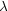<crit = 42 G/2, will be unstable. Unlike for the Jeans instability in a stationary medium, longer waves are stabilized by rotation, embodied in the2 term.

For disks with random motion, the reduction factor0 for large |k|, irrespective of the frequency, for the reason given above. Thus random motion stabilizes short waves, as for the Jeans instability. Toomre (1964) showed for a Gaussian distribution of radial velocities that the RHS of eq. (8) is0 for all |k| whenRR,crit, where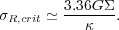(11)

Thus Toomre's local axisymmetric stability criterion is QR /R,crit1. When reasonably constant with radius, the locally estimated Q value is a good indicator of global axisymmetric stability. For example, the local values in the equilibrium models proposed by Kalnajs (1976) are in reasonable agreement with those he derived from global axisymmetric modes (his Fig. 2). It should be noted that an axisymmetrically stable disk, i.e. for which Q1 everywhere, could still be unstable to non-axisymmetric modes.

The local axisymmetric stability criterion for rotating gas disks is similar. The longest unstable wavelength,crit in a cold (vs = 0) disk is the same as for a stellar disk. The minimum of the quadratic expression in k on the RHS of eq. (10) occurs for k =G/ vs2, and the condition of marginal stability (2 = 0) at this minimum is readily solved, yielding vs,crit =G/.

Vandervoort (1970), Romeo (1992), and others have extended the discussion of axisymmetric stability to disks of finite thickness. The principal difference is the dilution of the self-gravity term caused by spreading the disk matter in the vertical direction, resulting in a somewhat more stable disk. The precise correction depends on the assumed vertical structure of both the disk and the wave, but is minor when the characteristic disk thickness z0 <<crit, which is usually the case.

More realistic composite disks consist of multiple stellar populations having a range of velocity dispersions, as well as a cool gas component. Jog & Solomon (1992) considered the stability of two gravitationally coupled gas disks having different sound speeds, but Rafikov (2001) improved their analysis to include multiple stellar components. These analyses show that while the gas component may contain a small fraction (typically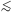10%) of the total mass, its low sound speed causes it to have a disproportionate effect on the overall stability.

Fig. 4 gives, for four different values of Q, a graphical representation of the stellar (solid curves), 3 and gaseous (dashed curves) dispersion relations eqs. (9) & (10) respectively. The curves do not differ for Q = 0 for which s is imaginary when k > kcrit. The dashed and solid curves have similar forms for moderate k but differ substantially for large k, where frequencies in the stellar sheet cannot exceed |- m| =. Thus waves in a stellar sheet extend only from CR, where s = 0, to the Lindblad resonance on either side, where s = 1. 4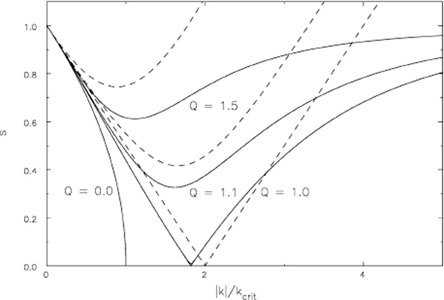Figure 4. The solid lines show the dispersion relation (9), while the dashed lines are from (10), each for several values of Q. The ordinate s = |- m| /. Since the signs of both the abscissa and the ordinate are suppressed, the graph is reflection symmetric about both axes. Note that s = 0 at CR and s = 1 at the Lindblad resonances.

A gas layer, on the other hand, can support gravitationally modified sound waves of arbitrarily high frequency. Note that this important difference from the stellar case can lead to artificial modes when hydrodynamic equations are used as a simplifying approximation for a stellar disk; while meaningful results can be obtained using this approximation, it is important to check that the derived modes do not cross Lindblad resonances.

In the quadrant shown, there are either two values of k for each value of s, known as the short- and long-wave branches, or there are none. Only for the marginally stable case of Q = 1 do solutions exist for all frequencies 0s1 and all |k|. When Q > 1, a forbidden region opens up in the vicinity of CR where waves are evanescent.

Recall that the relation (9) was derived assuming both |kR| >> 1 and that the waves are tightly wrapped - i.e. that the wave vector is directed radially. These approximations may not be too bad on the short-wave branch (large k) but long-wave branch solutions may not exist at all, except in a very low-mass disks where kcrit is large, and must fail as |k|0. Lynden-Bell & Kalnajs (1972) gave an improved dispersion relation (their eq. A11) that relaxed the requirement that the wave vector be radial, but still used the plane wave potential (7) that requires |kR| >> 1. Their relation is no different for tightly-wrapped waves, but has no long-wave branch or forbidden region for open waves when Q1.

The waves in a disk described by the local dispersion relations (9) & (10) have a phase speed, equal to the pattern speed, in the azimuthal direction. However, Toomre (1969) pointed out that these dispersive waves also have a group velocity that is directed radially. Since vg =/k, the group velocity is proportional to the slope of the lines in Fig. 4. Each portion of a wavetrain, or wave packet, is carried radially at the group velocity, retaining its pattern speedwhilst gradually adjusting its wavelength, and also transporting wave action or angular momentum.

For trailing waves outside CR, the situation illustrated in Fig. 4, the group velocity is positive on the short-wave branch, and the waves carry angular momentum outwards towards the OLR. The curves have the opposite slope inside CR, where density waves are disturbances of negative angular momentum (stars there lose angular momentum as the wave is set up, Lynden-Bell & Kalnajs 1972), and therefore the inward group velocity on the short-wave branch leads once more to outward angular momentum transport. Outward angular momentum transport is in the sense expected from the gravitational stresses of a trailing spiral (Lynden-Bell & Kalnajs 1972).

However, the sign of the slope/k on the dubious long-wave branch is opposite to that of short waves, indicating that angular momentum in that regime is transported in the direction opposite to the gravity torque! This apparent contradiction is resolved by the phenomenon of lorry transport, a term coined by Lynden-Bell & Kalnajs (1972) to describe an advective Reynolds stress (see also Appendix J of BT08). Stars in their epicyclic oscillations gain angular momentum from the wave near apo-center and lose it back to the wave near peri-center; no star suffers a net change, but angular momentum is still carried inward at a sufficient rate to overwhelm the gravity torque.

Thus where eq. (9) holds in a disk with approximately uniform Q1, a tightly wrapped trailing wave packet originating on the long-wave branch, will travel towards CR until it reaches the edge of the forbidden zone where it "refracts" into a short wave that carries it away towards the Lindblad resonance. The details of the turning point at the forbidden zone, which requires an evanescent wave propagating into the forbidden region, were described by Mark (1976) and by Goldreich & Tremaine (1978).

The fate of the short-wave propagating towards theLindblad resonance also requires more sophisticated analysis because the dispersion relation (9) does not hold near resonances. Mark (1974) carried the analysis to second order to show that the wave is absorbed there through the wave-particle coupling described by Lynden-Bell & Kalnajs (1972).

Goldreich & Lynden-Bell (1965b), Julian & Toomre (1966) and Toomre (1981) extended the above analysis for tightly-wrapped waves to waves of arbitrary inclination. They again adopted the approximate potential of a short-wavelength plane wave (eq. 7), and focused on a local patch of the disk whose dimensions were assumed small compared with R0, the radial distance of the patch center, which orbits the disk center at the local circular speed V = R00.

Following Hill (1878), they then introduced Cartesian-like coordinates, xR - R0 and yR0(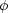-0), where0 is the azimuth of the orbiting center of the patch. The vertical coordinate, z, is unchanged from the usual cylindrical polar coordinate. Since the angular rotation rate decreases outwards in galaxies, the motion of stars in the patch is that of a shear flow, with a speed that varies as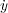= -2Ax, together with Coriolis forces arising from the rotation of the patch at angular rate0. The Oort "constant" A = -1/2 R d/ dR. The undisturbed surface density of matter,, is assumed constant over the patch. This set of approximations is described as the sheared sheet (see BT08 pp. 678-681).

Ifis the inclination angle of a plane density wave to the azimuthal direction, with 180° >> 90° for leading waves and 90° >> 0° for trailing waves, then the shear causes the pitch angle to change with time as cot= 2At, with t = 0 when= 90°, i.e. when the wave is purely radial. Both Goldreich & Lynden-Bell (1965b) for a gaseous sheet and Julian & Toomre (1966) for a stellar sheet found waves amplify strongly, but transiently, as they shear from leading to trailing.

The unremitting shear flow ultimately tears apart any transient disturbance, and indeed Julian & Toomre (1966) asserted that the stellar sheet is locally stable at small-amplitudes when Q1, but they did not give the proof.

Fig. 5, from Toomre (1981), illustrates the dramatic transient trailing spiral that results from a small input leading disturbance. This calculation illustrates that even a global, small-amplitude disturbance does not persist, but decays after its transient flourish. In the late stages, the "wave action" propagates at the group velocity (Section 3.5) away from corotation, where it is absorbed at the Lindblad resonances.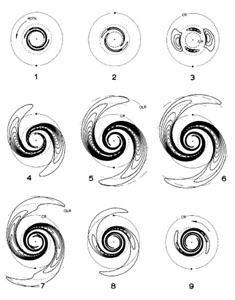Figure 5. The time evolution of an input leading wave packet in the half-mass Mestel disk. The unit of time is half a circular orbit period at the radius marked CR. Reproduced from Toomre (1981).

Notice also that the wave in this Q = 1.5 disk extends across the forbidden region near CR. The absence of solutions near s = 0 when Q > 1 in Fig. 4 is a consequence of assuming a steady, tightly-wrapped wave; shearing waves do not respect this restriction.

The responsiveness of a disk to input leading signal depends both on the degree of random motion - it decreases rapidly over the range 1 < Q < 2 - and upon the azimuthal wavelength, which isy = 2R / m for an m-armed pattern. Julian & Toomre (1966) defined the parameter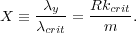(12)

In a disk having a flat rotation curve, Toomre (1981) showed that the strongest amplification occurs when 1X2.5, or about twice the scale of the longest unstable axisymmetric wave. The peak amplification shifts to larger X values for declining rotation curves and vice versa for rising ones. Naturally, the phenomenon of swing amplification disappears entirely in uniformly rotating disks.

By making the assumption that spiral patterns in galaxies are amplified solely by this mechanism, Athanassoula et al. (1987) argued that the multiplicity of the observed spiral arm pattern can be used to place bounds on the mass density in the disk. The circular orbit speed in a nearly axisymmetric galaxy affords a direct estimate of the central attraction, but it is hard to determine how much of the total attraction is contributed by the disk, and how much by other components, such as the dark matter halo. Becausecrit is proportional to the disk surface density, for a given rotation curve, the most vigorous amplification shifts to higher sectoral harmonics in lower mass disks. Generally, disks that contribute most of the central attraction, i.e. close to maximum disks, would prefer bi-symmetric spirals, while higher arm multiplicities would indicate significantly sub-maximal disks (see also Sellwood & Carlberg 1984).

2 Goldreich & Lynden-Bell (1965a) derived the vertically-integrated equations for "2D pressure" in a gas disk. Back.

3 The reduction factor used assumes a Gaussian distribution of radial velocities. Back.

4 Additional Bernstein-type waves exist near integer values of s > 1, but such solutions seem to be of little dynamical importance. Back.